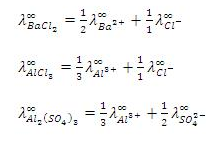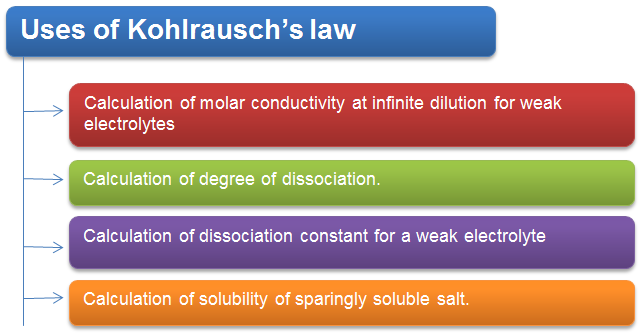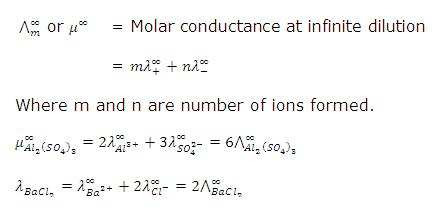Click to Chat

1800-1023-196

+91-120-4616500

CART 0

• 0

MY CART (5)

Use Coupon: CART20 and get 20% off on all online Study Material

ITEM
DETAILS
MRP
DISCOUNT
FINAL PRICE
Total Price: Rs.

There are no items in this cart.
Continue ShoppingKohlrausch’s law

Table of Content

Kohlrausch’s Law States that

Uses of Kohlrausch’s law

Calculation of Molar Conductivity at Infinite Dilution For Weak Electrolytes

Calculation of Degree of Dissociation

Calculation of Dissociation Constant For a Weak Electrolyte

Calculation of Solubility of Sparingly Soluble Salt

Related Resources

Kohlrausch’s Law States that"At infinite dilution, when dissociation is complete, each ion makes a definite contribution towards equivalent conductance of the electrolyte irrespective of the nature of the ion with which it Is associated and the value of equivalent conductance at infinite dilution for any electrolyte is the sum of contribution of its constituent ions", i.e., anions and cations. Thus,

where,

According to Kohlrausch’slaw. “conductivity of ions is constant at infinite dilution and it does not depend on nature of co-ions.”For AxBy type electrolyte,

Here Z+and Z- are the charges present on cation and anion.

Here m and n are the number of moles of cations and anions.Uses of Kohlrausch’s lawCalculation of Molar Conductivity at Infinite Dilution For Weak Electrolytes

As already mentioned, the molar conductivity  of weak electrolytes at infinite dilution cannot be determined experimentally, firstly because the conductance of such a solution is low and secondly because dissociation of such an electrolytes is not completed even at high dilutions.

The molar conductivity of such an electrolyte at infinite dilution can be calculated using Kohlrausch’s lawCalculation of Degree of Dissociation

According to Arrhenius theory of electrolytic dissociation, the increase in the molar conductivity with dilution is entirely due to the increase in the dissociation of the electrolyte; the molar conductivity at infinite dilution being maximum because the dissociation is almost complete.

Thus if  is the molar conductivity of a solution at any concentration C and  the molar conductivity at infinite dilution (i.e. zero concentration), we will have

However, this relationship is found to hold good only for weak electrolytes. The value of  for the weak electrolytes can be calculated , using Kohlrausch’s law, as discussed already in the first application.

Calculation of Dissociation Constant For a Weak Electrolyte

Knowing the degree of dissociation (as calculated above) the dissociation constant (K) of the weak electrolyte at concentration C of the solution can be calculated

using the formula

Calculation of Solubility of Sparingly Soluble Salt

Salts such as AgCl. BaSO4, PbSO4 etc which dissolve to a very small extent in water are called sparingly soluble salts.

A they dissolve very little, their solutions are considered as infinitely dilute. Further as their solutions are saturated, their concentration is equal to their solubility.

Thus by determining the specific conductivity (K) and the molar conductivity of such solutions , we have

Solved Example

Question:

From the given molar conductivities at infinite dilution, calculate  for NH4OH.

for Ba(OH)2 = 457.6 ohm-1 cm2mol-1.

for Ba(Cl)2 = 240.6 ohm-1 cm2mol-1.

for NH4Cl = 129.8 ohm-1 cm2mol-1.

Solution:

Ba(OH)2 =  + ..(i)

(BaCl2 )=  + ...(ii)

(NH4Cl) =  + ..(iii)

f (NH4OH) = +

= 1/2 eq. (i) + eq (iii) -1/2 eq (ii)

=

= 238.3 ohm-1 cm2 mol-1Question 1:

According to Kohlrausch’s law, conductivity of ions is constant at

a. fixed temperature

b. infinite dilution

c. 1 M concentration

d. all the concentrations

Question 2:

According to Kohlrausch’s law, conductivity of ions at infinite dilutions does not depend on

a. nature of co-ions

b. temperature

c. pressure

d. concentration

Question 3:

Which of the following salts is not sparingly soluble one?

a. NaCl

b. AgCl.

c.  BaSO4

d. PbSO4

Question 4:

What would be conductivity of MX2 if conductivity of X- and M2+ is 5 ohm-1cm2mol-1 and 6 ohm-1cm2mol-1 respectively?

a. 10 ohm-1cm2mol-1

b. 11 ohm-1cm2mol-1

c.14 ohm-1cm2mol-1

d.17 ohm-1cm2mol-1Q.1

Q.2

Q.3

Q.4

b

a

a

d

Related Resources

Refer to the  Syllabus of IIT JEE

Look into the Past Year Papers of IIT JEE

You can also refer to Electrochemical Series

To read more, Buy study materials of Electrochemistry comprising study notes, revision notes, video lectures, previous year solved questions etc. Also browse for more study materials on Chemistry here.### Course Features

• 731 Video Lectures
• Revision Notes
• Previous Year Papers
• Mind Map
• Study Planner
• NCERT Solutions
• Discussion Forum
• Test paper with Video Solution